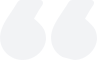# Calculation formula for hedging positions

2023-08-02Summary:

Hedging positions aim to reduce overall risk by simultaneously holding related but opposite assets, which can offset certain adverse market fluctuations and protect the investment portfolio from losses. This strategy can help investors mitigate risks and stabilize investment returns when the market is uncertain.

Hedging positions are an investment strategy aimed at reducing the overall risk of an investment portfolio by simultaneously holding interrelated but opposite assets or securities. The purpose of this strategy is to reduce potential losses by spreading the risks in the investment portfolio across multiple asset classes.The main idea of hedging positions is to establish short-term opposite investment positions on one or more assets or securities, which means that if one position experiences losses, the other position will be compensated. For example, investors can purchase a certain stock and corresponding options simultaneously, so that even if the stock price drops, the options may generate returns to offset stock losses.

By hedging their positions, investors can protect their investment portfolio from market fluctuations. This strategy is suitable for investors who want to maintain stable appreciation of their funds rather than pursue high-risk and high returns.

Hedged positions are typically used in the following areas:

1. Directional hedging

Investors own a certain asset or position and wish to hedge the price risk associated with that asset or position. They will establish trades in the opposite direction to reduce the impact of market volatility on their investment portfolio.

2. Option hedging

Investors purchase or sell option contracts and establish corresponding hedging positions to reduce losses caused by fluctuations in option contract prices. This hedging can be dynamic and needs to be adjusted based on changes in option prices and portfolio values.

3. Cross-variety hedging

Investors establish positions in opposite directions between different markets or asset classes to offset price risks in different markets. For example, an oil company may purchase crude oil futures contracts to hedge its crude oil price risk while establishing transactions in the opposite direction to hedge the price risk of its related products.

Calculation formula for hedging positions

The calculation formula for hedging positions can vary depending on the actual situation of the investment portfolio, but one common calculation method is to determine hedging positions by calculating the hedging ratio.

The hedging ratio refers to the proportion of hedging assets held by investors in hedging positions. A common method of calculating hedge ratios is to use the beta coefficient, which is a measure of the correlation between assets and the market.

Firstly, it is necessary to calculate the correlation between the investment portfolio and the hedged assets. This can be achieved by calculating the covariance between the investment portfolio and the hedged asset. Covariance measures whether the changing trends of two variables are consistent, that is, whether their changes are in the same direction.

Then, calculate the variance of the investment portfolio and hedging assets. Variance measures the magnitude of a variable's fluctuation, that is, its degree of volatility.

Next, use the values of covariance and variance to calculate the hedge ratio. The calculation formula for the hedging ratio is as follows:

Hedge Ratio=Covariance (Portfolio, Hedge Asset)/Variance (Hedge Asset)

Among them, Covariance (Portfolio, Hedge Asset) represents the covariance between the investment portfolio and the hedged asset, and Variance (Hedge Asset) represents the variance of the hedged asset.

The calculated hedging ratio can be used to determine the weight of hedging positions. For example, if the hedging ratio is 0.5, it means that investors need to allocate 50% of their funds to hedge assets and the remaining 50% to other assets in the investment portfolio.

The calculation formula for hedging positions can vary depending on specific circumstances, and the accuracy of hedging ratios depends on the stability of correlations. More details and complex models may be required to adapt to actual situations, and hedging may not always completely eliminate risks.### What is the status of the volume-price relationship?

The volume-price relationship is a key stock market indicator, revealing the correlation between trading volume and stock prices. Analyzing these changes helps investors understand market activity and potential trend reversals.

2023-12-01### What is delisting?

Delisting removes a stock from public trading. It's either voluntary or mandatory, due to violations, financial issues, mergers, etc.

2023-12-01### What does the exchange rate mean?

The exchange rate, reflecting the relative values of two currencies, is influenced by factors like currency supply and demand, balance of payments, economic growth rate, interest rates, monetary policy, and inflation.

2023-11-29